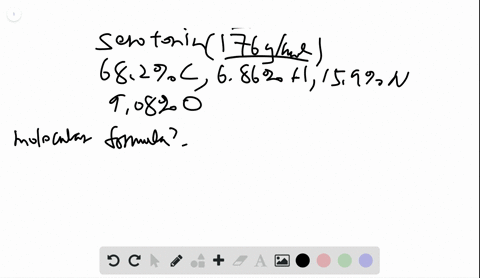Enroll in one of our FREE online STEM bootcamps. Join today and start acing your classes!View Bootcamps### Serotonin $(M=176 \mathrm{g} / \mathrm{mol})$ tra…

02:49University of Maine
Problem 101

## Discussion

You must be signed in to discuss.

## Video Transcript

to find the percent hydrogen in a given compound. We look at the formula and then the amount of hydrogen in one mole of the compound over the overall molar mass of the compound. And so it's simply the number of hydrogen ins times the molar mass of hydrogen, which is 1.8 divided by the molar mass of the compound. And then, because it's a percentage, we multiply by 100. So for each of these compounds, we first need to find the formulas. Methane is a hydrocarbon. C two h six propane IHS C three h eight Another hydrocarbon. Benzene is also hydrocarbons C six h six. Ethanol is an alcohol compound see to H five h and then finally were given the formula for fetal palm imitate. We just see 32 h 64. Oh, too reach compound. We can then find the percent hydrogen For the first compound. There are six hydrogen ins times the molar mass of hydrogen over the total molar mass of the compound, which is to times the molar mass of carbon, plus six times the molar mass of hydrogen. We're six 0.0 for eight, divided by 30 0.7 So in this compound, 20% hydrogen in propane, we have eight hydrogen times the molar mass of hydrogen divided by the total Mueller Mass, which is three times 12.11 for carbon, plus eight times the molar mass of hydrogen. And these numbers are all found on the periodic table, and so, in propane we have 18%. Hydrogen bending is very similar. The mass of hydrogen divided by the total more mass when we find that bendy, this 7.7 percent ethanol has six. Hydrogen is total, and we can add those. Hydrogen is together divided by the molar mass below or two times the carbons, plus six times the hydrogen, plus the one oxygen for 13.1%. And finally, for acetyl palm it eight. There are 64 hydrogen ins over a total of 32 carbons, plus the 64 hydrogen plus two oxygen's or 64 0.512 over 480 0.85 or 13.4%. So we can see that this compound effin has the greatest amount, and benzine has the least and arranging from highest to lowest percentage received. The ethane is greater than propane, which is greater than the Seattle Paul imitate, which is greater than ethanol, which is greater than benzene.